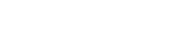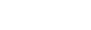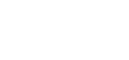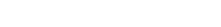# Mathematics Paper 2 Questions and Answers - Form 4 End Term 2 2021

MATHEMATICS
PAPER 2
TIME: 2 ½ HOURS

INSTRUCTIONS

• This paper consist of TWO sections: section I and Section II.
• Answer ALL the questions in Section I and only five questions from section II.
• Show all the steps in your calculations, giving your answers at each stage in the stage in the spaces below each question.
• Marks may be given for correct working even if the answer is wrong.
• Non-programmable silent electronic calculators and KNEC mathematical tables may be used, except where stated otherwise.

SECTION I : 50 MARKS.

1. Evaluate without using Mathematical tables or a calculator. (3mks)
2log 5 - 1/2log 16 + log40
2. The sum of K terms of sequence 3,9,15,21............is 7500. Determine the value of K. (3mks)
3. Use matrix method to solve (3mks)
5x +3y =35
3x -4y = -8
4. Calculate the percentage error in the volume of a cone whose radius is 9.0cm and slant length 15.0cm. (3mks)
5. Make y the subject the subject of the formula (3mks)
p/w = (my-2)/(ny+4)
6. Solve for x: tan2 x – 2 tan x = 3 for the interval 0 ≤x≤ 180o (3 marks)
7. The table below shows income tax rates in the year 2013.
 Monthly Income in Ksh Tax rate in each shilling Up to 9680 10% 9681-18800 15% 18801 – 27920 20% 27921 – 37040 25% Over 37040 30%
In that year, a monthly personal tax relief of ksh 1056 was allowed. Calculate the monthly income tax by a constable who earned a monthly salary of ksh. 42500 (3mks)
8. Simplify leaving your answer in the form a+b√c, where a, b and c are rational numbers. (3mks)9.
1. Expand (1-n)5 (2mks)
2. Use the expansion in (a) up to the term in n3 to approximate the value of (0.98)5 (2mks)
10. The probability that three candidates; Anthony, Beatrice and Caleb will pass an examination are 3/42/3 and 4/5 and respectfully. Find the probability that:-all the three candidates will not pass. (2mks)
11. The equation of a circle is X2 + Y2 -4x +6y + 4 = 0. On the graph provided draw the circle (4mks)
12. Find the shortest distance between points A(50oS,25ot) and B(50oS, 140oE in KM (Take R=6370 Km) (3mks)
→
13. The mid-point of AB is (1,-1.5, 2) and the position vector of a point A is -1+j is . Find the magnitude of AB correct to 1dp. (3mks
14. Without using a calculator or mathematical tables. Express in surd form and simplify (3mks)
3
1-COS 300
15. The figure below shows a circle centre O. AB and PQ are chords intersecting externally at a point C. AB = 9cm, PQ= 5cm and QC = 4cm. Find the length of BC. (3mks)16. Evaluate without using tables
Log(3x+8) -3log2 = log(x-4) (4mks)

SECTION II (50 MARKS)
Answer ONLY FIVE questions in this section

1.
1. Use the trapezium rule with six trapezia to excrete the areas bounded by the curve Y=2n2+ 3n +1, the axis and the ordinate x=0 and x=3. (5mks)
2. Calculate the exact axed in (a) above by integration. (3mks)
3. Assuming they are calculated in (a) above is an estimate, calculate the percentage error made when the trapezium rule is used leaving your answer to 2 decimal places. (2mks
2. In the diagram below <EDG=360 and <ABG=420 Line EDC and ABC are tangents to the circle at D and B respectively.Calculate by giving reason
1. <DGB (2mks)
2. Obtuse <DOB (2mks)
3. <GDB (2mks)
4. <DCB (2mks)
5. <DFB (2mks
3. The table below shows the rate at which income tax is charged for all income earned in a month in 2015.
Taxable Income p.m (Kenya pound) Rate in % per Kenya pound
1 -236 10%
237 -472 15%
473 -708 20%
709 – 944 25%
945 and over 30%
Mrs.mumanyi earns a basic salary of 18000.She is entitled to a house allowance of Ksh. 6,000 a person relief of Ksh. 1064 month
Every month she pays the following.
1. Electricity bill shs.580
2. Water bill shs. 360
3. Co-operative shares shs. 800
4. Loan repayment Ksh. 3000
1. Calculate her taxable income in k£ p.m (2Marks)
2. Calculate her P.A.Y.E (6Marks)
3. Calculate her net salary (2Marks)
4. A flower garden is in the shape of a triangle ABC such that AB = 9M, AC=7.5M and angle ACB=75%. Using a rule and a pair of compass only.
1. Construct ΔABC (3mks)
2. Construct a locus of P such that AP = pc (2mks
3. Construct locus of Q such that it is equal distance from AB and BC and locus of R which is 2M from AC. (2mks)
4. Flowers are to be planted such that they are nearer AC than AB and less than 5m from a shade the portion with flowers. (3mks)
5. A tank has two water taps P and Q and another tap R. When empty the tank be filled by tap P alone in 5 hours or by tap Q in 3 hours .When full the tank can be emptied in 8 hours by tap R
1. The tank is initially empty . Find how long it would take to fill up the tank
1. If tap R is closed and taps P and Q are opened at the same time (2mks)
2. If all the three taps are opened at the same time .Giving your answer to the nearest minute (2mks)
2. Assume the tank initially empty and the three taps are opened as follows
P at 8:00 am
Q at 9:00 am
R at 9:00 am
1. Find the fraction of the time that would be filled by 10:00 am (3mks)
2. Find the time the tank would be fully filled up. Give your answer to the nearest minute (3mks)
6. The figure below shows a cuboid.Calculate
1. The length BE (2Mks)
2. The angle between BE and plane ABCD (3Mks)
3. The angle between FH and BC. (2Mks)
4. The angle between place AGHD and plane ABCD. (3Mks)
7. In triangle OAB below OA = a, OB = b point M lies on ON such that OM : MA= 2:3 and point N lies on OB such that ON: NB = 5:1 line AN intersect line MB at X.1. Express in terms of a and b
1. AN (1mk
2. BM (1mk)
2. Given that AX=kAN and BX=rBM where k and r are scalars.
1. write down two different expression for OX in terms of a, b, k and r. (2mks)
2. Find the value of k and r. (4mks)
3. Determine the ratio in which x divides line MB. (2mks)
8.
1. Complete the table below for the function y=n3-3k2-k+2 for -2 ≤n ≤4. (2mks)
 X -2 -1 0 1 3 4 Y -6 2 14
2. On the grid provided, draw the graph of y =n3-3n2-n+2. (3mks)
3. Use the graph to solve the equation
n3 -3n2 – x + 2 = 0 (2mk)
4. By drawing a suitable line on the graph, solve the equation n3- 3n3 – 3n + 3 = 0 (3mks)

MARKING SCHEME

1.Log (25x1600/4)
Log 10,000
4 log 10
=4
2. n=? δn=n/2 (2a+(n-1)d)
a=3
d=6
δn=7500
7500=n/2 [2(3)+(n-1)6]
7500=n/2 [6+6n-6]
7500=n/2(6n)
7500=3n2
n2=2500
n=50
3.4. 8.95 – 9.05
14.5 – 15.5
Max=1/3 x 22/7 x 9.052  x 15.5=132.94
Min = 1/3  x 22/7 x 8.92 x 14.5=1216.80
Actual = 1/3 x 22/7 x 92 x 15=1272.86
A.E = 1/2(1329.94-1216.80
=56.57
%error = 56.57/1272.86 x 100
=4.445%
5. P/w = (my-2)/(ny+4)
Pny + 4p = mwy – 2w
4p+2w = mwy – pny
4p +2w = (mw – pn) y
y= (4p+2w)/(mw-pn)
6. tan2x – 2 tan x -3=0
Let tan x be P
P2-2p -3=0
P(p-3) + 1(p-3)=0
(p-3)(p+1)=0
P=3 or p=-1
tan x =3
x=71.57o or x = 135o
7. 1st tax band = 9680 x 10/100 = 968
2nd tax band = 9120 x 15/100 = 1368
3rd tax band = 9120 x 20/100 = 1824
4th tax band = 9120 x 25/100 = 2280
5th tax band = 5460 x 30/100 = 1638
Gross tax = 8078..............................................
Relief = 1056
Net tax = 7022
Monthly income tax = 7022
8.9.

1.  X5 15 14 13 12 11 10 Y 0 (-n)1 (-n)2 (-n)3 (-n)1 (-n)5 coeff 1 5 10 10 5 1 1 -5n -10n2 -10n3 5n4 -n5
(1-n)5=1-5n+10n2-10n2+5n4-n5
2. (0.98)5 = (1-0.02)5 = (1-n)5
= 0.02=n
1-5(0.02) + 10(0.02)2 -10(0.02)3
1-0.01+0.004-0.00008 = 0.99392
10. P(fail) = 1/2  x 1/3 x 1/5 = 1/60
11. x2+y2-4x+6y+4=0
x2-4x+y2+6y=-4
(x2-4x + (-2)2+(y2+6y+(3)2=-4+(-2)2+(3)2
(x-y)2+(y+3)2=9
Centre =(2,-3)
12. A(50oS, 25oE)
B(50oS, 140oE) θ= 140-25
long
θ, ∝ θ=115o
km =θ/360 2π2
115 o x 2 x 22/7 x 6370
360
= 12,790.56km
13.= √(4+6.25+4)
= √14.25
= 3.775 x 2
= 7.550
=7.6
14. Cos 30º = √3/2
3/1-cos 30º =     3
1- 3/2
=6/2-√3
=6/2-√3 x (2+√3)/(2+√3) = 12+6√3/4+2√3 - 2√3 -3
15. y(y+9) = 4x9
y2+9y-36=0
y2 + 12y-3y-36=0
y(y+12)-3(y+12)=0
(y-3) (y-12) = 0...................................................
Y=3 or y=-12(ignore)
∴ = BC = 3cm
16. Log (3x+8) – lof 8 = 10g (x – 4)
Log (3x+8/8) = log (x-4)drop logs
3x+8/8 x – 4
3x+8=8x-32
3x – 8x = -32 -8
-5x = -40
X=8
17.

1.  X 0 0.5 1 1.5 2 2.5 3 Y 1 3 6 10 15 21 28
A= ½ h[(yo + yn) + 2(y1 +y2+y3+y4+y5)]
= ½ (0.5) [(1+28) + 2(3+6+10+25+21)]
= ¼ x 139
= 34.75
2. 03(2x2 + 3x+)dx
=[2x3 + 3x2 + x (30)
3       2=2/3 X 27 + 3/2 X 9+ 3
=18/1 + 27/2 + 3/1
=(36+27+6)/2
69/2
= 34.5
3. Error = actual estimate
34.5 – 34.75
= -0.25
%error = - 0.25/34.5 x 100
= 0.725 decrease
18.
1. <DGB=78º(Angles alternate segment are equal subtended by the same chord)
2. Obtuse <DOB = 156º subtended at the centre by BD is twice the angles subtended at the circumference by BD
3. <GDB= 54º (<chord and tangent)
4. <DCB=180º(2x78)=24º
angles in  a triangle add up to 180º
5. <DFB=180º-78º=102º
opposition angle in a cyclic
19.
1. T.T = (18000+6000)/20
= £ 1200 p.m
2. 1st 236 x 10/100 = 23.6
2nd 236 x 15/100 = 35.4
3rd 236 x 20/100 = 47.2
4th 236 x 25/100 = 59
20.

1.2. Constructing bisector of line AC
locating locus P
3. Bisecting angle ABC (locus Q)
constructing a line 2cm away from AC locating locus R
4. Bisecting < BAC
drawing an arc (dotted) 5cm away from A.
5. Tap P in 1 hour = 1/5 of tank
Tap Q in 1 hour= 1/3 of tank
Tap R empties= 1/8 of tank
21.
1.
1. 1/5+1/3= 8/15
Time = 1 ÷ 8/15
= 1 x 15/8 = 1.875 hour
= 1 hour 53 min
2. 1/5+1/3-1/8 = 24+40-15 =   49
120         120
Time= 1 ÷ 49/120
=1 x 120/49= 2.44898
=2hrs 27 min
2.
1. Pin 1 hr = 1/5
in 2hrs = 2 x 1/5 = 2/5
Q in 1hr = 1/3
1/3 x 1= 1/3
R=1/8 x 1
Fractional filled = 2/5 + 1/4 -1/8 = 73/100
2. in 1 hr= 49/120
x= 47//120
x= 47/120 x 120/49 = 0.9592=58 min
22.
1. SOH CAH TOA
H2= 82 + 62
=64+36= 100
√100=10
2. Tan θ= 5/10
θ = tan-1 0.5=26.56
3. Tan θ = 8/6
θ= tan-1 1.333
=53.12
4. Tan θ = 5/8= 0.625
θ=tan-1 0.625=32
23.
1.
1. AN= AO + ON
= -a + 5/6 B
= -a + 5/6 b
2. BM=BO+OM
= -b + 2/5OA
= -b + 2/5a
2.
1. OX= OB +BX
=b + rBM
=b+ r(b+2/5a)
=b-rb +2/5ra
(1-r)b + 2/5ra

OX=OA+AX
=OA + kAN
= a + k(-a + 5/6b)
=a - ka + 5/6kb
=(1-k)a + 5/6kb

2. OX = OX
=(1-r)b + 2/5ra=(1-k)a+ 5/6 kb
(1-r)b = 5/6kb
1-r = 5/6k
2/5ra = (1-k)a
2/5r = 1-k
r=5/2(1-k)
=5/2 -5/2k
1-5/6k= 5/2 - 5/2k
-5/6k + 5/2k= 5/2 -1
-5k+15k = 5-2
6             2
10k/6 = 3/2
k= 3/2 x 6/10 = 9/10
r=1-5/6k
1-5/6(9/10)
1-3/4
=1/4
3. BX = rBM
BX= 1/4BM
BX/BM = 1/4
MX:XB
3:1
24.
1.
 X -2 -1 0 1 2 3 4 Y -16 -1 2 -1 -4 -1
2. Graph drawn3.
1. y = n3 - 3n2 - n + 2
o= n3 - 3n2 - n + 2
y=0
x = 0.8 ± 0.05 or 0.9 ± 0.05 0r 3.1 ± 0.05
2. y = n3 - 3n2 - n + 2
o= n3 - 3n2 - 3n + 3
y= 2n-1
 x 0 1 y -1 1
k = -1.1 ± 0.05 or 0.7 ± 0.05 or 3.6 ± 0.05

• ✔ To read offline at any time.
• ✔ To Print at your convenience
• ✔ Share Easily with Friends / Students

.
Subscribe now

access all the content at an affordable rate
or
Buy any individual paper or notes as a pdf via MPESA
and get it sent to you via WhatsApp